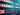# Superdense codingSuperdense coding is a protocol that, in some sense, achieves a complementary aim to teleportation. Rather than allowing for the transmission of one qubit using two classical bits of communication (at the cost of one e-bit of entanglement), it allows for the transmission of two classical bits using one qubit of quantum communication (again, at the cost of one e-bit of entanglement).

In greater detail, we have a sender (Alice) and a receiver (Bob) that share one e-bit of entanglement. According to the conventions in place for the lesson, this means that Alice holds a qubit , Bob holds a qubit , and together the pair is in the state Alice wishes to transmit two classical bits to Bob, to be denoted by and , and she will accomplish this by sending him one qubit.

It is reasonable to view this feat as being less interesting than the one that teleportation accomplishes. Sending qubits is likely to be so much more difficult than sending classical bits for the foreseeable future that trading one qubit of quantum communication for two bits of classical communication, at the cost of an e-bit no less, hardly seems worth it. However, this does not imply that superdense coding is not interesting, for it most certainly is.

Fitting the theme of the lesson, one reason why superdense coding is interesting is that it demonstrates a concrete and (in the context of information theory) rather striking use of entanglement. A famous theorem in quantum information theory, known as Holevo's theorem, implies that without the use of a shared entangled state, it is impossible to communicate more than one bit of classical information by sending a single qubit. (Holevo's theorem is more general than this. It's precise statement is technical and requires explanation, but this is one consequence of it.) So, through superdense coding, shared entanglement effectively allows for the doubling of the classical information-carrying capacity of sending qubits.

Another reason why superdense coding is interesting is because of its close, complementary relationship with teleportation, which will be discussed further at the end of the section.

XPERIMEN.COM